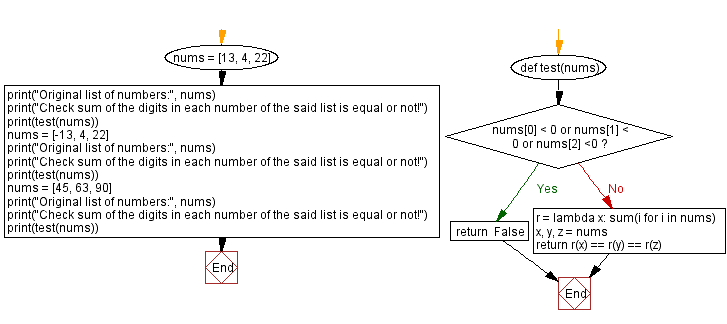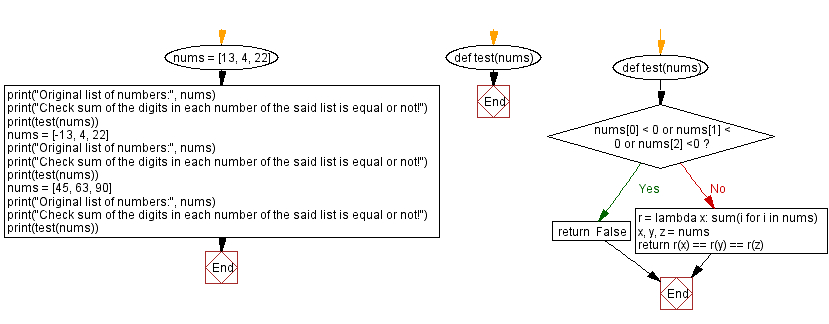﻿ Python - Sum of the digits in each number in a list is equal

# Python Exercises: Sum of the digits in each number in a list is equal

## Python Basic - 1: Exercise-147 with Solution

A Python list contains three positive integers. Write a Python program to check whether the sum of the digits in each number is equal or not. Return true otherwise false.

Sample Data:

([13, 4, 22]) -> True
([-13, 4, 22]) -> False
([45, 63, 90]) -> True

Sample Solution-1:

Python Code:

``````def test(nums):
return nums % 9 == nums % 9 == nums % 9

nums = [13, 4, 22]
print("Original list of numbers:", nums)
print("Check sum of the digits in each number of the said list is equal or not!")
print(test(nums))
nums = [-13, 4, 22]
print("Original list of numbers:", nums)
print("Check sum of the digits in each number of the said list is equal or not!")
print(test(nums))
nums = [45, 63, 90]
print("Original list of numbers:", nums)
print("Check sum of the digits in each number of the said list is equal or not!")
print(test(nums))
``````

Sample Output:

```Original list of numbers: [13, 4, 22]
Check sum of the digits in each number of the said list is equal or not!
True
Original list of numbers: [-13, 4, 22]
Check sum of the digits in each number of the said list is equal or not!
False
Original list of numbers: [45, 63, 90]
Check sum of the digits in each number of the said list is equal or not!
True
```

Flowchart:Sample Solution-2:

Python Code:

``````def test(nums):
if nums < 0 or nums < 0 or nums <0:
return  False
r = lambda x: sum(i for i in nums)
x, y, z = nums
return r(x) == r(y) == r(z)

nums = [13, 4, 22]
print("Original list of numbers:", nums)
print("Check sum of the digits in each number of the said list is equal or not!")
print(test(nums))
nums = [-13, 4, 22]
print("Original list of numbers:", nums)
print("Check sum of the digits in each number of the said list is equal or not!")
print(test(nums))
nums = [45, 63, 90]
print("Original list of numbers:", nums)
print("Check sum of the digits in each number of the said list is equal or not!")
print(test(nums))
``````

Sample Output:

```Original list of numbers: [13, 4, 22]
Check sum of the digits in each number of the said list is equal or not!
True
Original list of numbers: [-13, 4, 22]
Check sum of the digits in each number of the said list is equal or not!
False
Original list of numbers: [45, 63, 90]
Check sum of the digits in each number of the said list is equal or not!
True
```

Flowchart:Python Code Editor:

Have another way to solve this solution? Contribute your code (and comments) through Disqus.

What is the difficulty level of this exercise?

Test your Programming skills with w3resource's quiz.

﻿